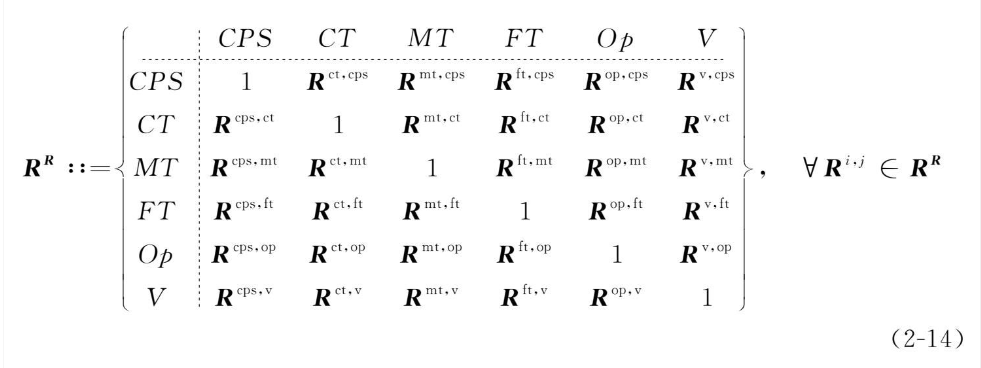2021-08-08 22:37

# 这种类型的Latex公式怎么写，求解• 好问题 提建议
• 收藏

#### 1条回答默认 最新

•八云黧 2021-08-08 23:10
已采纳

没全给出来，简写了一部分，但用到的语法都在里面了

$$R^R::=\left \{ \begin{array}{c:ccc} &CPS&CT&MT&FT&Op&V \\ \hdashline CPS & 1&R^{ct,cps} &R&R&R&R \\ CT & R&1&R&R&R&R \\ MT & R&R&1&R&R&R \\ FT & R&R&R&1&R&R \\ Op & R&R&R&R&1&R \\ V & R&R&R&R&R&1 \\ \end{array} \right \}, \forall R^{i,j} \in R^R$$

已采纳该答案
评论
解决 无用
打赏 举报